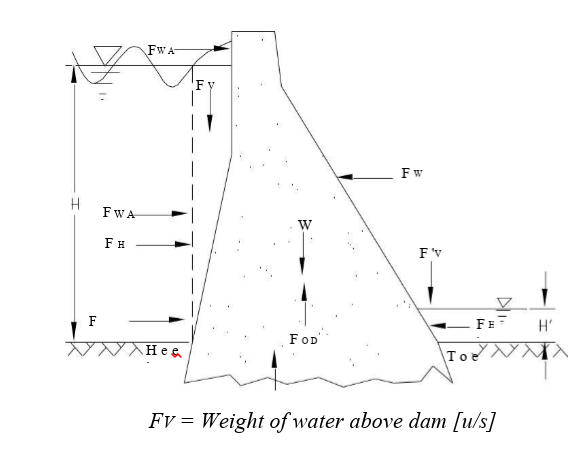### Concrete Gravity Dam

Introduction

• Basically, gravity dams are solid concrete structures that maintain their stability against design loads from the geometric shape and the mass and strength of the concrete. Generally, they are constructed on a straight axis, but may be slightly curved or angled to accommodate the specific site conditions.
• Gravity dams typically consist of a non-overflow section(s) and an overflow section or spillway.

Forces acting on Gravity Dams

• The structural integrity of a dam must be maintained across the range of circumstances or events likely to arise in service. The design is therefore determined through consideration of the corresponding spectrum of loading conditions. In all foreseeable circumstances the stability of the dam and foundation must be ensured, with stresses contained at acceptable levels and watertight integrity essentially unimpaired.

​​​​​​​Representation of typical loads acting on Gravity dam

Where:

depth H’ = Tail Water depth FWA

= Wave pressure force

FH = Horizontal hydrostatic force

FS = Silt/sediment pressure force

FEQ = Earthquake/Seismic force F W = Wind pressure force FH’ = Tail water hydrostatic force W = Weight of dam

FOD = Internal pore water pressure

FU = Uplift pressure force [base of dam] F U  FV = Weight of water above dam [u/s]

FV’  = Weight of water above dam [d/s]